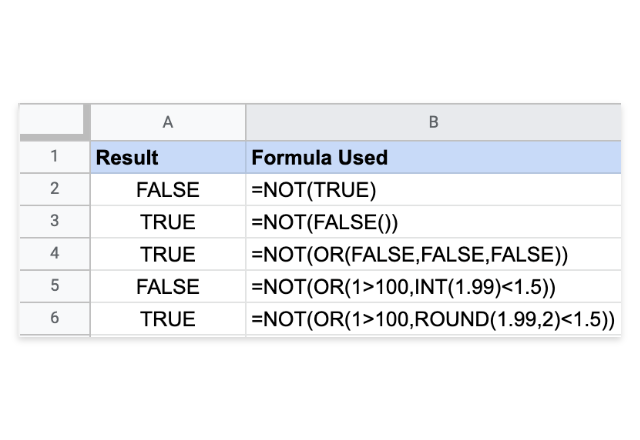# How to use the FALSE formula in Google SheetsThe FALSE formula in Google Sheets returns the logical value – FALSE. Perhaps, it is one of the most simplest and straightforward formulas. It is the opposite of the TRUE formula in Google Sheets, which returns the logical value – TRUE.

### Syntax

FALSE()

Please note that the formula requires no input arguments.

In most of the scenarios, Google Sheets converts the literal text FALSE to the logical FALSE. So, to input the logical FALSE in a formula, we just have to key in the word FALSE directly and Google Sheets will interpret this as the logical FALSE.

Also, apart from using this formula directly to generate the logical FALSE value, the Google Sheets application can do this in many different ways. Some of the examples are shown in the snapshot below.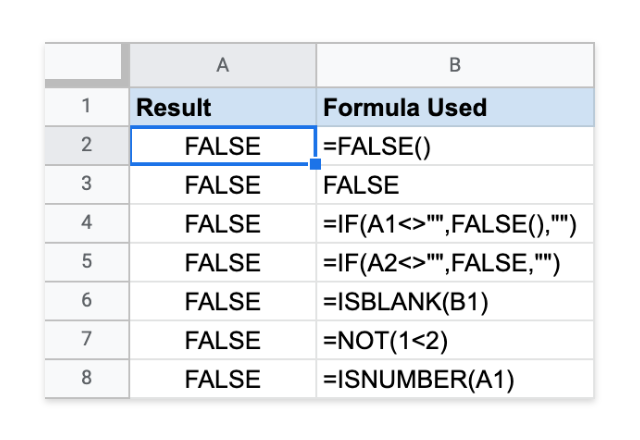### Usage: FALSE Formula

As a standalone formula, FALSE() may not have many practical uses. But, its presence is quite ubiquitous. Because the logical expressions that return logical values form the cornerstones of many conditional formulas. Without the interplay between the logical expressions and the logical TRUE and FALSE values, many important formulas are as good as nothing. In the examples below, the conditional expressions enable the formulas to consider only the TRUE scenarios and ignore the ones that are FALSE.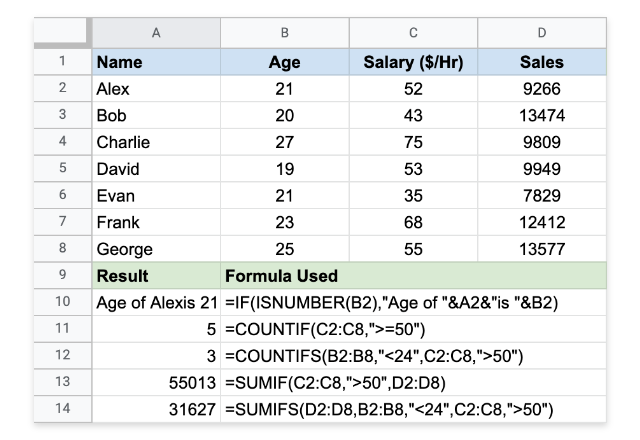##### FALSE Formula with AND, OR, and NOT

While we established that the logical TRUE and FALSE values are founding pillars on which many other formulas build upon, we need to understand how the logical formulas AND, OR and NOT work with the logical TRUE and FALSE values.

##### AND Formula

This returns a logical TRUE if we set every input parameter value to logical TRUE. If we set any of the parameters to a logical FALSE, then, as a result, the output value is a logical FALSE.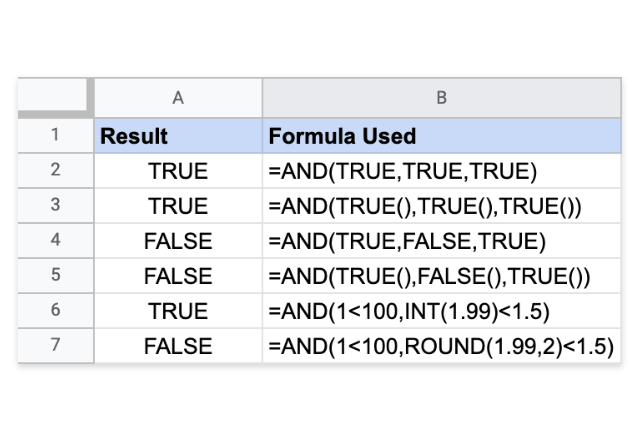##### OR Formula

This returns a logical TRUE if we set any of the input parameter values to logical TRUE. Therefore, if we set every input parameter to logical FALSE, then the output value is a logical FALSE.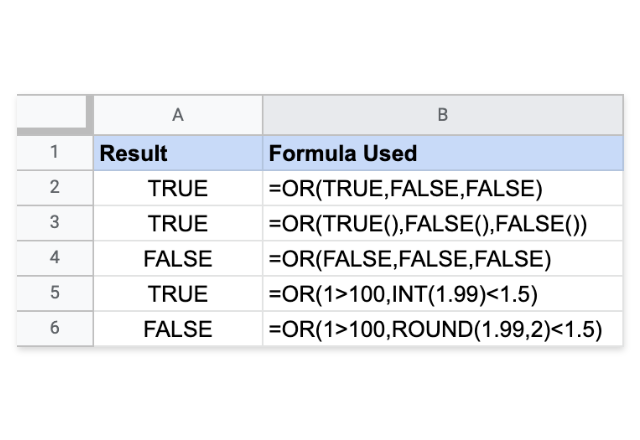##### NOT Formula

This returns a logical TRUE if we set the input parameter to logical FALSE, and vice versa. Hence, the output is just the negation of the input logical value.